• 表示随机现象（在一定条件下，并不总是出现相同结果的现象称为随机现象）各种结果的... 随机变量X是定义在基本空间Ω上的取值为实数的函数，即基本空间Ω中每一个点，也就是每个基本事件都有实轴上的点与之对应。例如
表示随机现象（在一定条件下，并不总是出现相同结果的现象称为随机现象）各种结果的变量（一切可能的样本点）。例如某一时间内公共汽车站等车乘客人数，电话交换台在一定时间内收到的呼叫次数等等，都是随机变量的实例。　　一个随机试验的可能结果（称为基本事件）的全体组成一个基本空间Ω 。 随机变量X是定义在基本空间Ω上的取值为实数的函数，即基本空间Ω中每一个点，也就是每个基本事件都有实轴上的点与之对应。例如，随机投掷一枚硬币 ，可能的结果有正面朝上 ，反面朝上两种 ，若定义X为投掷一枚硬币时正面朝上的次数
， 则X为一随机变量，当正面朝上时，X取值1；当反面朝上时，X取值0。又如，掷一颗骰子 ，它的所有可能结果是出现1点、2点、3点、4点、5点和6点 ，若定义X为掷一颗骰子时出现的点数，则X为一随机变量，出现1，2，3，4，5，6点时X分别取值1，2，3，4，5，6。　　要全面了解一个随机变量，不但要知道它取哪些值，而且要知道它取这些值的规律，即要掌握它的概率分布。概率分布可以由分布函数刻画。若知道一个随机变量的分布函数，则它取任何值和它落入某个数值区间内的概率都可以求出。　　有些随机现象需要同时用多个随机变量来描述。例如 ，子弹着点的位置需要两个坐标才能确定，它是一个二维随机变量。类似地，需要n个随机变量来描述的随机现象中，这n个随机变量组成n维随机向量 。描述随机向量的取值规律 ，用联合分布函数。随机向量中每个随机变量的分布函数，称为边缘分布函数。若联合分布函数等于边缘分布函数的乘积
，则称这些单个随机变量之间是相互独立的。独立性是概率论所独有的一个重要概念。 　　random variable 　　在不同的条件下由于偶然因素影响，其可能取各种不同的值，具有不确定性和随机性，但这些取值落在某个范围的概率是一定的，此种变量称为随机变量。随机变量可以是离散型的，也可以是连续型的。如分析测试中的测定值就是一个以概率取值的随机变量，被测定量的取值可能在某一范围内随机变化，具体取什么值在测定之前是无法确定的，但测定的结果是确定的，多次重复测定所得到的测定值具有统计规律性。随机变量与模糊变量的不确定性的本质差别在于，后者的测定结果仍具有不确定性，即模糊性。

展开全文• 定义 1.3.1： 设设设 EEE 为一个随机试验，其样本空间为一个随机试验，其样本空间为一个随机试验，其样本空间 S={e},X=X(e)S=\{e\},X=X(e)S={e},X=X(e) 及及及 Y(e)Y(e)Y(e) 是定义在 SSS 上的两个随机变量，由她们...
1.3 多维随机变量及其分布

一、二维随机变量

定义 1.3.1：  $设$ $E$ $为一个随机试验，其样本空间$ $S=\{e\},X=X(e)$ $及$ $Y(e)$ 是定义在 $S$ $上的两个随机变量，由她们构成的联合随机变量$ $(X,Y)$ $称为二维随机变量或二维随机向量。$
分布函数： $设$ $(X,Y)$ $是定义在$ $S上的二维随机变量，对于任意实数$ $x,y,$ $二元函数：$
$F(x,y) = P\{X \leq x,Y \leq y\}$

例题1：  将 $P\{x_1 转换为分布函数表达式

答案：
$F(x_2,y_2)-F(x_1,y_2)-F(x_2,y_1)+F(x_1,y_1)$

1. 二维分布函数的性质：

$F(x,y)$ $是变量$ $x$ $和$ $y$ 的不减函数，即
$\forall x_1 \leq x_2,F(x_1,y) \leq F(x_2,y)$$\forall y_1 \leq y_2,F(x,y_1) \leq F(x,y_2)$
$0 \leq F(x,y) \leq 1$，且对固定的 $x$，$F(x,-\infty) = 0$且对固定的 $y$，$F(-\infty,y)=0$ 且$F(- \infty,-\infty)=0$$F(+ \infty,+\infty)=1$
$F(x,y)$ 关于变量 $x$ 和 $y$ 均是右连续的，即 $\forall x,y$ 有 $F(x+0,y)=F(x,y)=F(x,y+0)$
对于任意的 $x_1 \leq x_2,y_1 \leq y_2$，下述不等式成立：$F(x_2,y_2)-F(x_1,y_2)-F(x_2,y_1)+F(x_1,y_1) \geq0$

2. 二维离散型随机变量：

定义 1.3.3： 若二维随机变量 $(X,Y)$ 的所有可能取值是有限对或可列多对：$(X,Y)=(x_i,y_i) \space\space\space i,j=1,2....$
满足以下性质：

$p_{ij} \geq 0 \space\space\space\space i,j=1,2....$
$\sum_{i=1}^{\infty}\sum_{j=1}^{\infty}p_{ij}=1$

分布函数： $F(x,y)=P\{X \leq x,Y\leq y\} = \sum_{x_i \leq x}\sum_{y_j \leq y}p_{ij}$
X的边缘分布律： $p_{i.}=P\{X=x_i\}=\sum_{j=1}^{\infty}p_{ij} \space\space\space i=1,2...$
X的边缘分布函数： $F_X(x)=F(x,+\infty)=\sum_{x_i \leq x}\sum_{y_j \leq +\infty}p_{ij}=\sum_{x_i \leq x}(\sum_{j=1}^{\infty}p_{ij})=\sum_{x_i\leq x}p_{i.}$
同理：
Y的边缘分布律： $p_{.j}=P\{Y=y_j\}=\sum_{i=1}^{\infty}p_{ij}\space\space\space j=1,2...$
Y的边缘分布函数： $F_Y(y)=F(+\infty,y)=\sum_{x_i \leq +\infty}\sum_{y_i \leq y}p_{ij}=\sum_{y_j \leq y}(\sum_{i = 1}^{\infty}p_{ij})=\sum_{y_j\leq y}p_{.j}$

例题2：

补满下表

x\y
1
2
3
$p_{i.}$

1
0
$\frac{1}{6}$
$\frac{1}{12}$

2
$\frac{1}{6}$
$\frac{1}{6}$
$\frac{1}{6}$

3
$\frac{1}{12}$
$\frac{1}{6}$
0

$p_{.j}$

x\y
1
2
3
$p_{i.}$

1
0
$\frac{1}{6}$
$\frac{1}{12}$
$\frac{1}{4}$

2
$\frac{1}{6}$
$\frac{1}{6}$
$\frac{1}{6}$
$\frac{1}{2}$

3
$\frac{1}{12}$
$\frac{1}{6}$
0
$\frac{1}{4}$

$p_{.j}$
$\frac{1}{4}$
$\frac{1}{2}$
$\frac{1}{4}$
1

根据上表求 $F_X(2),F_Y(3)$

$F_X(2)=\frac{3}{4},F_Y(3)=1$

3. 二维连续型随机变量：
定义1.3.5： $设二维随机变量$ $(X,Y)$ $的分布函数为$ $F(x,y)$ $若存在一个非负可积函数的二元函数$ $f(x,y)$，$使它对于任意实数$ $x,y$ 都有 $F(x,y)=\int_{-\infty}^x \int_{-\infty}^y f(x)dxdy$
$则称$ $(X,Y)$ $为二维连续随机变量$，$函数$ $f(x,y)$ $称为二维随机变量$ $(X,Y)$ 的概率密度，或称为随机变量 $X$与 $Y$ 的联合概率密度。
满足以下性质：

$f(x,y) \geq 0 \space\space\space -\infty < x,y < +\infty$
$\int_{-\infty}^{+\infty}\int_{-\infty}^{+\infty}f(x)dxdy=1$
若 $f(x,y)$ 在点 $(x,y)$ 连续，则$\frac{\delta^2F(x,y)}{\delta x\delta y}=f(x,y)$
随机点 $(X,Y)$ 落在平面区域 $D$ 内的概率 $P\{(x,y)\in D\}={\int\int}_Df(x,y)dxdy$

例题3： 已知随机变量 $X$ 和 $Y$ 的联合概率密度为 $f(x,y)=\begin{Bmatrix}Ce^{-(2x+y)} \space\space\space x>0,y>0 \\ 0 \space\space\space 其他 \end{Bmatrix}$
(1) 试求 $C$ 的值
(2) $P\{X

答案：
(1)
由 $1=\int_{-\infty}^{+\infty}\int_{-\infty}^{+\infty}f(x,y)dxdy=\int_{0}^{+\infty}\int_{0}^{+\infty}Ce^{-(2x+y)}dxdy=$ $C\int_{0}^{+\infty}e^{-2x}dx\int_{0}^{+\infty}e^{-y}dy=C(-\frac{1}2{}e^{-2y}|_{0}^{+\infty})(-e^{-y}|_{0}^{+\infty})=\frac{C}{2}$ 得 $C=2$ 即 $f(x,y)=\begin{Bmatrix}2e^{-(2x+y)} \space\space\space x>0,y>0 \\ 0 \space\space\space 其他 \end{Bmatrix}$
(2)
$P\{X $2\int_{0}^{+\infty}e^{-2x}dx \space* (-e^{-y})|_{x}^{+\infty}=2\int_{0}^{+\infty}e^{-2x}dx \space * e^{-x}=2\int_{0}^{+\infty}e^{-3x}dx=2(-\frac{1}{3}e^{-3x})|_{0}^{+\infty}=\frac{2}{3}$

$X$ 边缘概率密度函数：$f_X(x)=\int_{-\infty}^{+\infty}f(x,y)dy$
故 $X$ 的边缘分布函数 $F_X(x)=\int_{-\infty}^{x}f_X(x)dx$
同理：
$Y$ 边缘概率密度函数：$f_Y(y)=\int_{-\infty}^{+\infty}f(x,y)dx$
故 $Y$ 的边缘分布函数 $F_Y(y)=\int_{-\infty}^{y}f_Y(y)dy$

二. 条件分布

1. 条件分布律
$P\{Y=y_j|X=x_i\}=\frac{P\{X=x_i,Y=y_j\}}{P\{X=x_j\}}=\frac{p_{ij}}{pi.}=p_{j|i}\space\space\space j=1,2...$
$P\{X=x_i|Y=y_j\}=\frac{P\{X=x_i,Y=y_j\}}{P\{Y=y_j\}}=\frac{p_{ij}}{p.j}=_{i|j}\space\space\space i=1,2...$
例题4： 设 $(X,Y)$ 的概率分布如下表，试求 $X=2$ 的条件分布律

x\y
0
1
2

-1
$\frac{1}{10}$
$\frac{1}{20}$
$\frac{7}{20}$

2
$\frac{3}{10}$
$\frac{1}{10}$
$\frac{1}{10}$

答案：

首先计算 $X=2$ 的边缘分布律为 $\frac{1}{2}$。
当 $X=2$ 的条件分布律为 $P\{Y=0|X=2\}=\frac{\frac{3}{10}}{\frac{1}{2}}=\frac{3}{5},P\{Y=1|X=2\}=\frac{\frac{1}{10}}{\frac{1}{2}}=\frac{1}{5},P\{Y=2|X=2\}=\frac{\frac{3}{10}}{\frac{1}{2}}=\frac{1}{5}$

1.4 随机变量的数字特征

一. 数学期望的概念

定义1.4.1： $设离散型随机变量$ $X$ $的分布律为$ $P\{X=x_k\}=p_k \space\space\space k=1,2...,$ $若级数收敛，则称$ $E(X)=\sum_{k=1}^{\infty}x_kp_k$ $为$ $X$ $的数学期望或概率均值，简称均值或期望$。
$\space\space\space\space$ $设连续型是随机变量$ $X$ 的概率密度为 $f(x)$，$若积分\int_{\infty}^{+\infty}xf(x)dx$ $绝对收敛$，$则称$ $E(X)=\int_{-\infty}^{+\infty}xf(x)dx$ $为连续型随机变量$ $X$ $的数学期望。$
$\space\space\space\space$ $若$ $X$ $的分布律为$ $P\{X=x_k\}=p_k \space\space\space k=1,2...,且\int_{k=1}^{+\infty}g(x_k)p_k$ $绝对收敛，则函数$ $Y=g(X)$ $的期望为$ $E(Y)=E[g(X)]=\sum_{k=1}^{\infty}g(x_k)p_k$ $其中$ $g$ $为连续函数$。
$\space\space\space\space$ $若连续型随机变量$ $X$ 的概率密度为 $f(x)$ $,且\int_{-\infty}^{+\infty}f(x)dx$ $绝对收敛，则函数$ $Y=g(X)$ $的期望为$ $E(Y)=E[g(X)]=\int_{-\infty}^{+\infty}g(x)f(x)dx$
数学期望的性质：

(线性法则)：$E(aX+b)=aE(X)+b$
(加法法则):  $E(X+Y)=E(X)+E(Y)$
(乘法法则)：当随机变量 $X,Y$ 相互独立时 $E(XY)=E(X)E(Y)$
(柯西-许瓦兹不等式)： $|E(XY)|^2 \leq E(X^2)E(Y^2)$

柯西-许瓦兹不等式证明过程

均值向量表示： $E(x)=avg(x)=\frac{1^Tx}{n}$
去均值向量： $\tilde{x} = x-avg(x)1$去均值向量的特性：$avg(\tilde{x})=0$

例题5： 设 $X$ 的分布律为

X
-2
-1
0
1

$p_k$
$\frac{1}{4}$
$\frac{1}{8}$
$\frac{1}{2}$
$\frac{1}{8}$

求 $Y=X^2 -1$ 的数学期望。

二. 方差的概念

定义1.4.2： $设$ $X$ $是一个随机变量，若$ $E\{[X-E(X)]^2\}$ $存在，则称$ $E\{[X-E(X)]^2\}$ $为$ $X$ $的方差，记做$ $D(X)$ $或$ $Var(X)$ $或$ $\sigma_x^2$ $。即$ $D(X)=Var(X)=E\{[X-E(X)]^2\}=\sigma_x^2$ $称$ $\sigma_x=\sigma(X)=\sqrt{D(X)}$ 为 $X$ $的标准差或均方差。$
由方差的性质易得：$D(X)=E(X^2)-[E(X)]^2$ $E(X^2)=D(X)+[E(X)]^2$

证明过程：$D(X)=E\{[X-E(X)]^2\}=E\{X^2-2XE(X)+[E(X)]^2\}$ 由期望的加法性质 $E(aX+b)=aE(x)+b$ 可得 $D(X)=E(X^2)-2E(X)E(X)+[E(X)]^2=E(X^2)-[E(X)]^2$
方差是反应数据疏散程度特征得量。方差大，说明数据疏散；方差小，说明数据集中。

方差的矩阵表示： $D(x)=\frac{\tilde{x}^T\tilde{x}}{n}=\frac{<\tilde{x},\tilde{x}>}{n}=\frac{||\tilde{x}||_2^2}{n}$

三. 协方差、相关系数

定义1.4.3： $设$ $(X,Y)$ $为二维随机变量，称$ $Cov(X,Y)=E[(X-E(X))(Y-E(Y))]$ $为$ $X$ $与$ $Y$ $的协方差$。
由方差和协方差性质易得 $D(X+Y)=D(X)+D(Y)+2Cov(X,Y)$ $Cov(X,Y)=E(XY)-E(X)E(Y)$

证明过程：

$Cov(X,Y)=E[(X-E(X))(Y-E(Y))]=E[XY-YE(X)-XE(Y)+E(X)E(Y)]=$ $E(XY)-E(Y)E(X)-E(X)E(Y)+E(X)(Y)=E(XY)-E(X)E(Y)$
$D(X+Y)=E[(X+Y-E(X+Y))^2]=E[(X-E(X)+Y-E(Y))^2]=$ $E[(X-E(X))^2+(Y-E(Y))^2+2(X-E(X))(Y-E(Y))]=$ $E[(X-E(X))^2]+E[(Y-E(Y))^2]-2E[(X-E(X))(Y-E(Y))]=D(X)+D(Y)-2Cov(X,Y)$

协方差得基本性质：

$Cov(X,Y)=Cov(Y,X)$
$Cov(aX,bY)=abCov(Y,X)$
$Cov(X_1\pm X_2,Y)=Cov(X_1,Y)\pm Cov(X_2,Y)$
$|Cov(X,Y)|\leq \sqrt{D(X)}\sqrt{D(Y)}$
$Cov(X,X)=D(X)$
若 $X$ 和 $Y$ 相互独立，则 $Cov(X,Y)=0$
$Cov(aX\pm b,cY\pm d)=acCov(X,Y)$

协方差是反映量随机变量 $X$ 和 $Y$ 相关关系的特征量，它与 $X$ 与 $Y$ 是同量纲的，而反应 $X$ 与 $Y$ 相关关系的无量纲的是相关系数。

协方差的矩阵表示： $Cov(a,b)=\frac{\tilde{a}^T\tilde{b}}{n}=\frac{<\tilde{a},\tilde{b}>}{n}$

定义1.4.4： $设$ $(X,Y)$ $为二维随机变量$，$D(X),D(Y),Cov(X,Y)$ $分别为$ $(X,Y)$ $方差与协方差，称$ $\rho_{XY}=\frac{Cov(X,Y)}{\sqrt{D(X)}\sqrt{D(Y)}}$ $为随机变量$ $X$ 与 $Y$ $相关系数。$
相关系数的性质：

$|\rho_{XY}|\leq 1$，$\rho$ 是一个表征 $(X,Y)$ 同线性相关紧密程度的量， $|\rho|$ 较大表示 $(X,Y)$ 线性相关程度较高，反之较低。
若 $(X,Y)$ 相互独立，且 $D(X),D(Y)>0$，则 $\rho_{XY}=0$。若 $(X,Y)$ 的相关系数 $\rho_{XY}=0$，则称 $X$ 与 $Y$ 不相关。

相关系数矩阵表示： $\rho_{ab}=\frac{\frac{\tilde{a}^T\tilde{b}}{n}}{\sqrt{{\frac{\tilde{a}^T\tilde{a}}{n}}}\sqrt{{\frac{\tilde{b}^T\tilde{b}}{n}}}}=\frac{\tilde{a}^T\tilde{b}}{\sqrt{\tilde{a}^T\tilde{a}}\sqrt{\tilde{b}^T\tilde{b}}}=\frac{\tilde{a}^T\tilde{b}}{||\tilde{a}||_2||\tilde{b}||_2}=\cos\angle(\tilde{a},\tilde{b})$展开全文• 文章目录二元随机变量，离散型随机变量分布律二元随机变量二元离散型随机变量（一）离散型随机变量的联合概率分布...设 X=X(e)X=X(e)X=X(e) 和 Y=Y(e)Y=Y(e)Y=Y(e) 是定义在 SSS 上的随机变量，由它们构成的向量 (X...
文章目录二元随机变量，离散型随机变量分布律二元随机变量二元离散型随机变量（一）离散型随机变量的联合概率分布律联合分布律的性质
二元随机变量，离散型随机变量分布律

二元随机变量

定义： 设 $E$ 是一个随机实验，样本空间 $S=\{e\}$；设 $X=X(e)$ 和 $Y=Y(e)$ 是定义在 $S$ 上的随机变量，由它们构成的向量 $(X,Y)$ 称为二维随机向量或二元随机变量。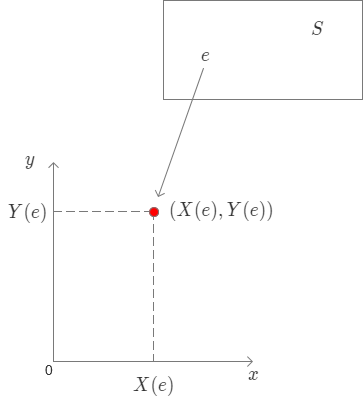二元离散型随机变量

定义： 若二元随机变量 $(X,Y)$ 全部可能取到的不同值是有限时或可列无线对，则称 $(X,Y)$ 是二元离散型随机变量。
（一）离散型随机变量的联合概率分布律
设 $(X,Y)$ 所有可能取值为 $(x_i,y_j)$，称 $P(X=x_i,Y=y_j)=P_{ij}，i,j=1,2,\cdots$ 为二元离散型随机变量 $(X,Y)$ 的联合概率分布律。也可简称 $(X,Y)$ 的分布律。可以用如下图的表格来表示
$\begin{array}{c|ccccc} _X\bcancel{\quad^Y} &y_1&y_2&\cdots&y_j&\cdots \\ \hline x_1 &p_{11}&p_{12}&\cdots&p_{1j}&\cdots \\ x_2 &p_{21}&p_{22}&\cdots&p_{2j}&\cdots \\ \vdots &\cdots&\quad&\cdots&\quad&\cdots \\ x_i &p_{i1}&p_{i2}&\cdots&p_{ij}& \cdots \\ \vdots &\cdots&\quad&\cdots&\quad&\cdots \end{array}$
联合分布律的性质

$p_{ij}\geq 0,$
$\sum_{i=1}^{\infty}\sum_{j=1}^{\infty}p_{ij}=1$
$P((X,Y)\in D)=\sum_{(x_i,y_j)\in D}p_{ij}$

其中 $p_{ij}=P(X=x_j,Y=y_j),i,j=1,2,\cdots$例 1： 一盒子中有 10 件产品，其中 6 件正品 ，4 件次品。从中取 1 件产品检验，不放回，再取 1 件检验。引入如下的随机变量 $X$ 与 $Y$，
$X=\begin{cases} 0, &\text{第 1 次取到次品} \\ 1, &\text{第 1 次取到正品}， \end{cases} \quad Y=\begin{cases} 0, &\text{第2次取到次品} \\ 1, &\text{第2次取到正品}, \end{cases}$
求 $(X,Y)$ 的联合分布律。
解： $(X，Y)$ 可能的取值数对有：$(0,0),(0,1),(1,0),(1,1).$
由乘法公式 $P(AB)=P(A)P(B|A)$ 得：
$P(X=0,Y=0)=P(X=0)P(Y=0|X=0)=\cfrac{4}{10}\times\cfrac{3}{9}=\cfrac{2}{15}$
同理得：$P(X=0,Y=1)=\cfrac{4}{10}\times\cfrac{6}{9}=\cfrac{4}{15}$
$P(X=1,Y=0)=\cfrac{6}{10}\times\cfrac{4}{9}=\cfrac{4}{15},P(X=1,Y=1)=\cfrac{6}{10}\times\cfrac{5}{9}=\cfrac{5}{15}$
$\begin{array}{c|cc} _X\bcancel{\quad^Y} & 0 & 1 \\ \hline 0 & \cfrac{2}{15} & \cfrac{4}{15} \\ 1 & \cfrac{4}{15} & \cfrac{5}{15} \end{array}$

例 2： 设随机变量 $X$ 在 1、2、3、4 四个正数中等可能地取一个值，另外一个随机变量 $Y$ 在 $1\sim X$ 中等可能地取一整数值，试求 $(X,Y)$ 的联合概率分布及 $X、Y$ 的分布。
解： $X、Y$ 的取值情况均为 1,2,3,4；当 $i,j=1,\cdots,4$ 时
$P(X=i,Y=j)=P(X=i)P(Y=j|X=i)=\begin{cases} \cfrac{1}{4}\times\cfrac{1}{i}, &i\geq j \\ \\ \cfrac{1}{4} \times 0, &i
联合概率分布律如下：
$\begin{array}{c|cc} _X\bcancel{\quad^Y} & 1 & 2 & 3 & 4 \\ \hline 1 & \cfrac{1}{4} & 0 & 0 & 0 \\ \\ 2 & \cfrac{1}{8} & \cfrac{1}{8} & 0 & 0 \\ \\ 3 & \cfrac{1}{12} & \cfrac{1}{12} & \cfrac{1}{12} & 0 \\ \\ 4 & \cfrac{1}{16} & \cfrac{1}{16} & \cfrac{1}{16} & \cfrac{1}{16} \end{array}$
求 $X、Y$ 分布律
$P(X=i)=1/4, i=1,2,3,4.$
$事件 \{X=1\},\cdots,\{X=4\}$ 是 $\{Y=j\}$ 前导事件组，由全概率公式得：
$P(Y=j)=\sum_{i=1}^{4}P(X=i)P(Y=j|X=i),j=1,2,3,4.$
所以，$X、Y$ 分布律就是在联合分布律表中横向、纵向相加！

例 3： 袋中有 1 个红球， 2 个黑球，3 个白球，现有放回地取两次，每次取一球，以 $X,Y,Z$ 分别表示两次取球所得的红、黑、白球个数。求：
（1）$P(X=1|Z=0)$
（2）$P(X=1,Z=0)$
（3）$(X,Y)$ 概率分布。
解：
（1） 这一问表示的意思是取到不是白球的前提下，取到 1 个红球的概率，所以：
$\quad P(X=1|Z=0)=\cfrac{1}{3}\times\cfrac{2}{3}+\cfrac{2}{3}\times\cfrac{1}{3}=\cfrac{4}{9}$
（2）这一问表达的是取出 1 个红球跟 0 个白球的概率，所以：
$\quad P(X=1,Z=0)=\cfrac{1}{6}\times\cfrac{2}{6}+\cfrac{2}{6}\times\cfrac{1}{6}=\cfrac{1}{9}$
这里需要注意两问的区别!
（3）$X，Y$ 的取值范围均为 0, 1, 2.
$P(X=0,Y=0)=\cfrac{3}{6}\times\cfrac{3}{6}=\cfrac{1}{4}\quad\quad$ 2 球均为白球
$P(X=0,Y=1)=\cfrac{2}{6}\times\cfrac{3}{6}\times2=\cfrac{1}{3}\quad\quad$ 黑白或者白黑
$P(X=1,Y=2)=0\quad\quad$ 这里总数超过 2 个，不符合条件。
$P(X=2,Y=0)=\cfrac{1}{6}\times\cfrac{1}{6}=\cfrac{1}{36}\quad\quad$ 两球均为红球
其余情况类似可得！
所以 $(X,Y)$ 的概率分布为：
$\begin{array}{c|cc} _X\bcancel{\quad^Y} & 0 & 1 & 2 \\ \hline 0 & \cfrac{1}{4} & \cfrac{1}{3} & \cfrac{1}{9} \\ \\ 1 & \cfrac{1}{6} & \cfrac{1}{9} & 0 \\ \\ 2 & \cfrac{1}{36} & 0 & 0 \\ \\ \end{array}$


展开全文• 随机变量是指变量的值无法预先确定仅以一定的可能性(概率)取值的量。 它是由于随机而获得的非确定值，是概率中的一个基本概念。 在经济活动中，随机变量是某一事件在相同的条件下可能发生也可能不发生的事件。 例如...
借鉴大佬的
下面附上网址
https://blog.csdn.net/ckk727/article/details/103435150
随机变量
随机变量是指变量的值无法预先确定仅以一定的可能性(概率)取值的量。
它是由于随机而获得的非确定值，是概率中的一个基本概念。
在经济活动中，随机变量是某一事件在相同的条件下可能发生也可能不发生的事件。
例如某一时间内公共汽车站等车乘客人数，电话交换台在一定时间内收到的呼叫次数等等，
都是随机变量的实例。
离散型随机变量
定义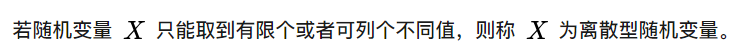分布函数性质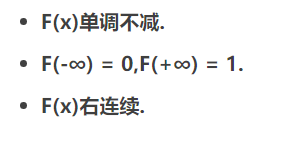个人觉得十分棒的图（思路很清晰）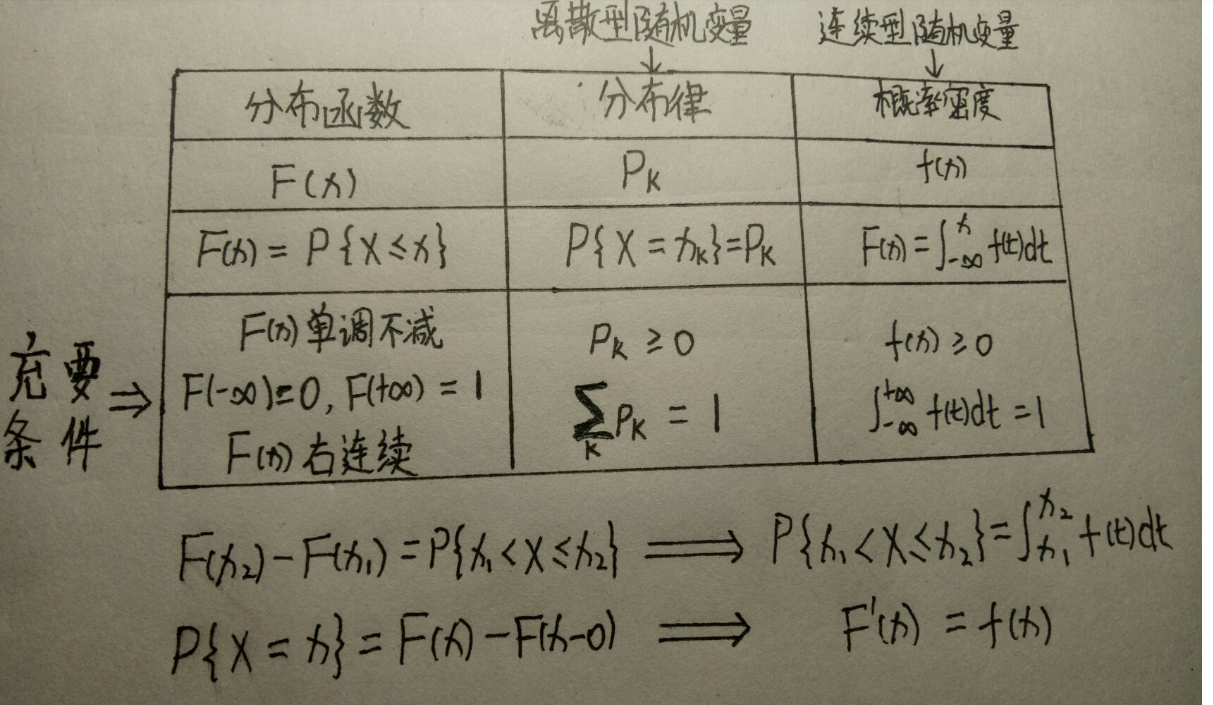离散型随机变量的常用分布
（1）0-1分布
（2）二项分布
（3）几何分布
（4）超几何分布
（5）泊松分布
连续型随机变量的常用分布
（1）均匀分布
（2）指数分布
（3）正态分布
详解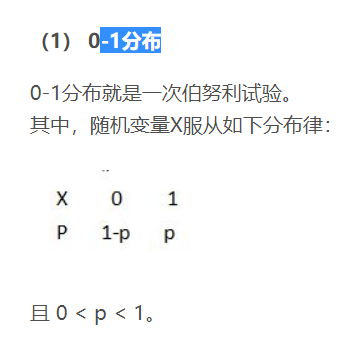核心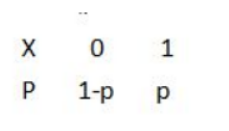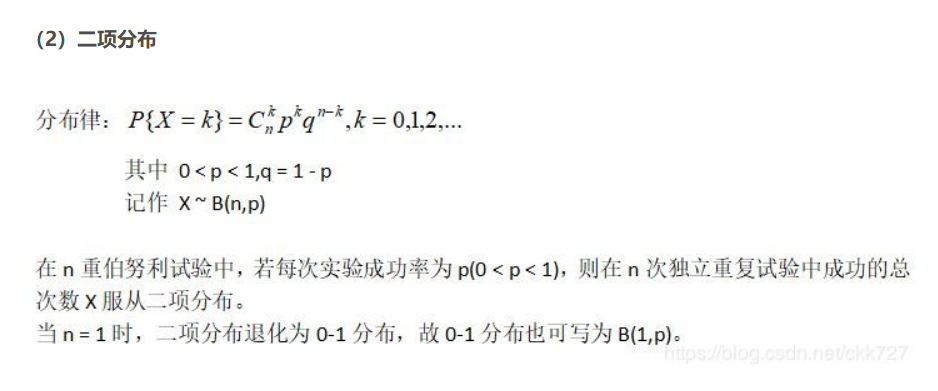核心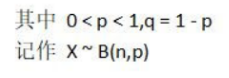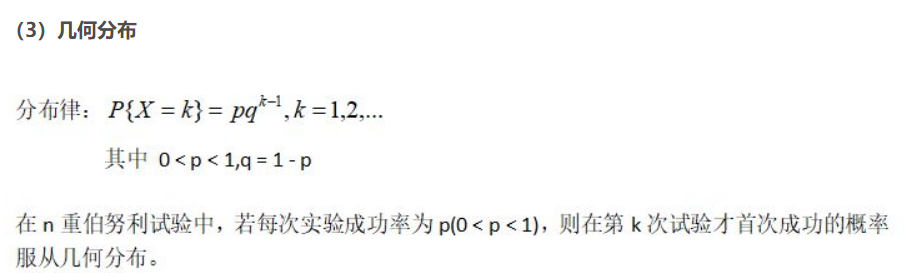核心（跟0-1分布差不多）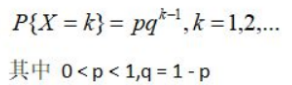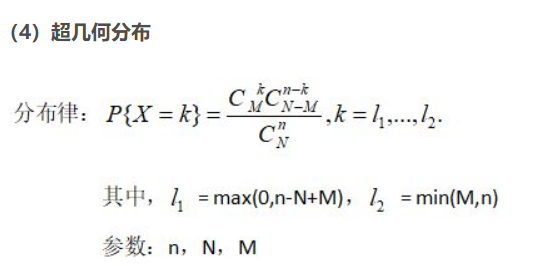核心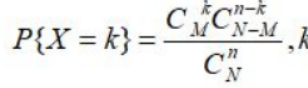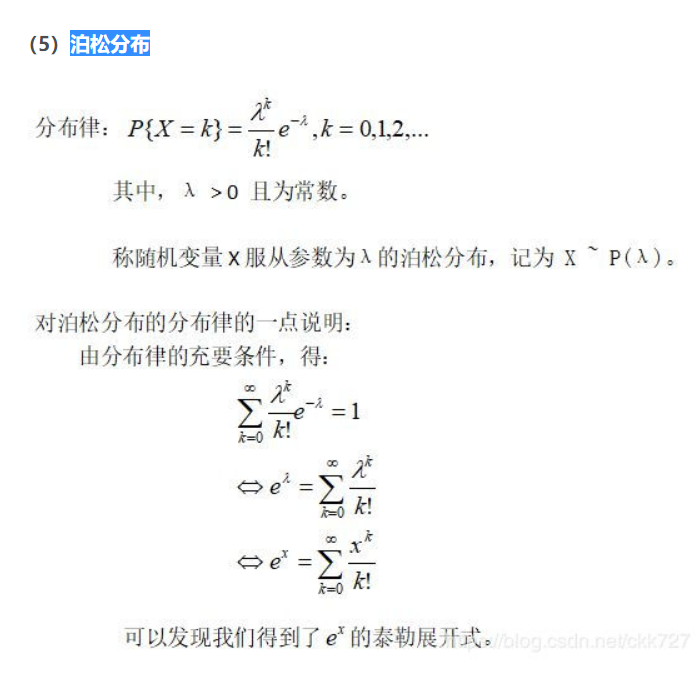核心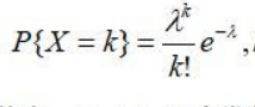连续型随机变量的常用分布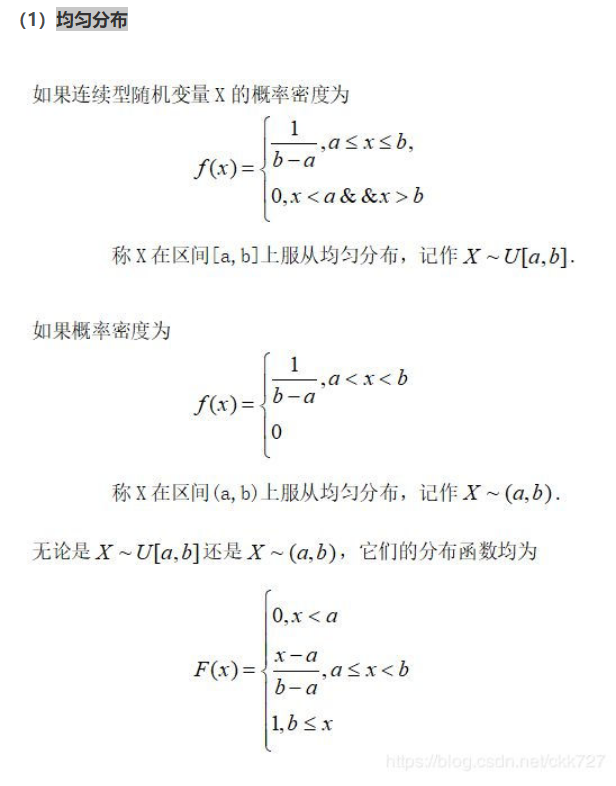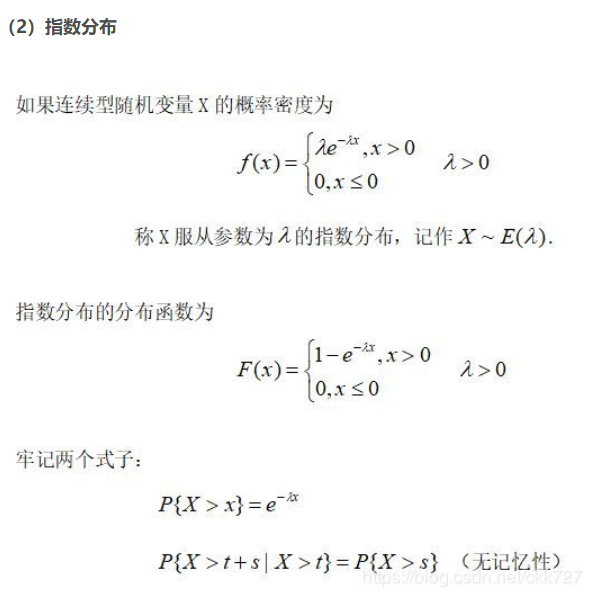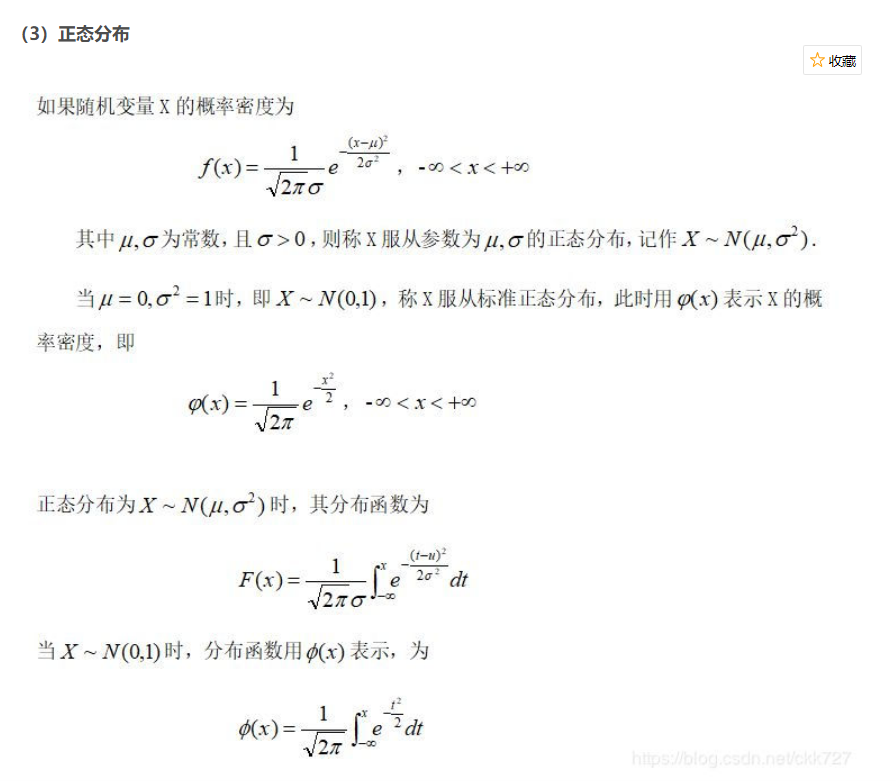补充
借鉴大佬
下面附上网址
https://blog.csdn.net/wangqingbang/article/details/91869130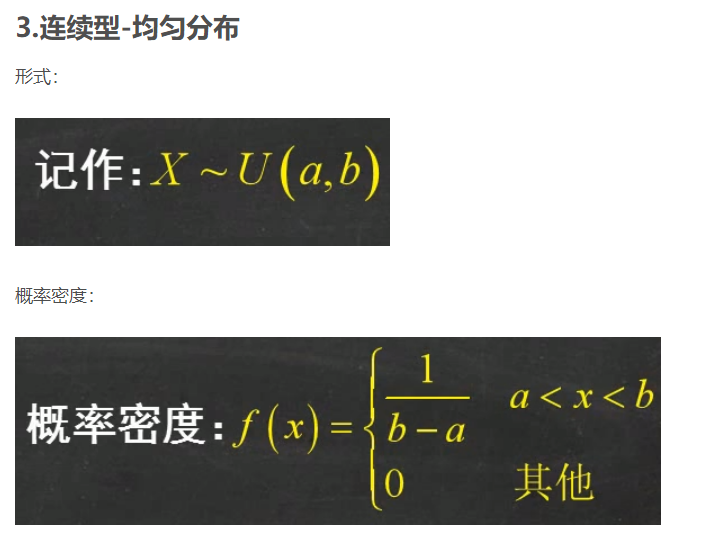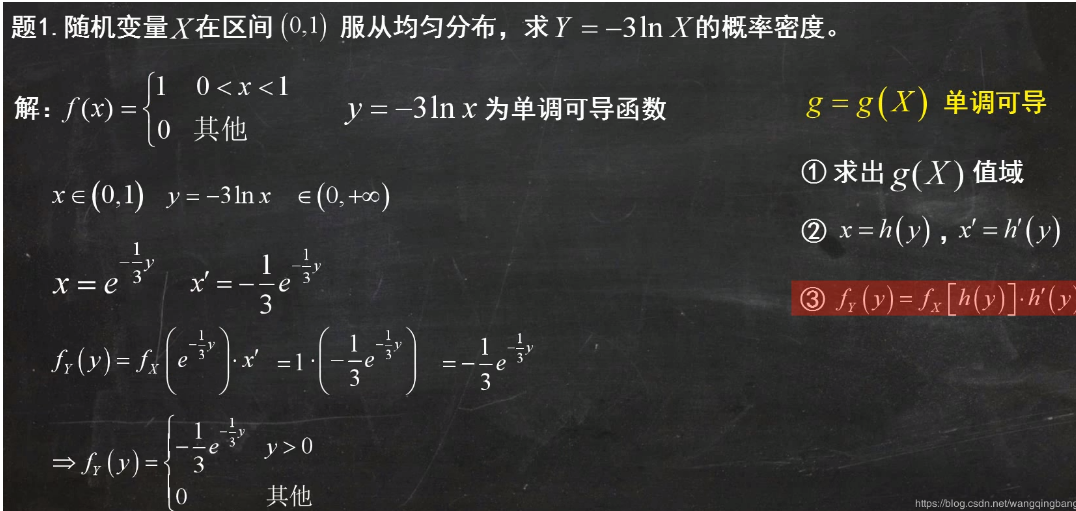讲的可详细了
我在这里补充一下
这是解题思路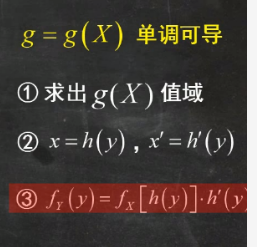x的值域，通过x的值域去求y的值域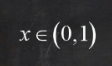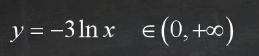调换顺序然后求导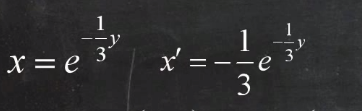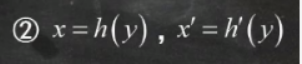他俩相等（我当时自己迷半天）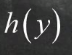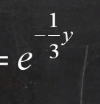最后用这个公式就好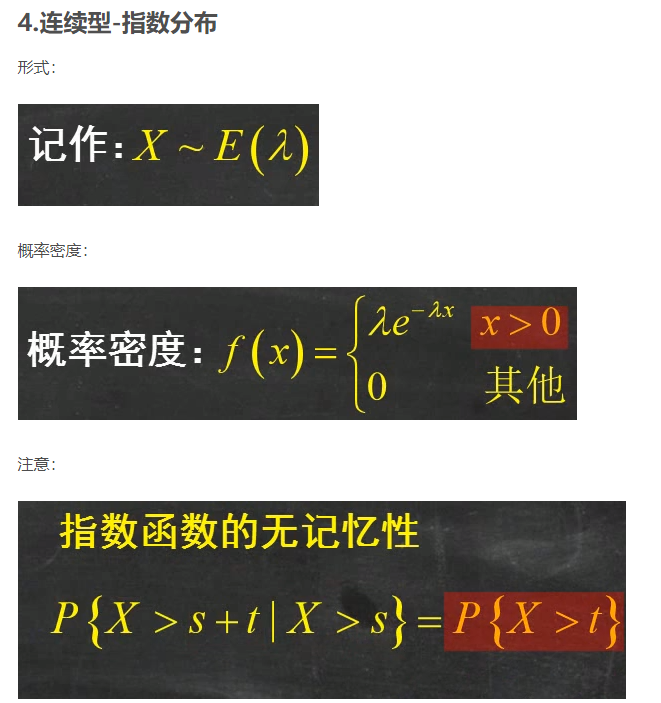例子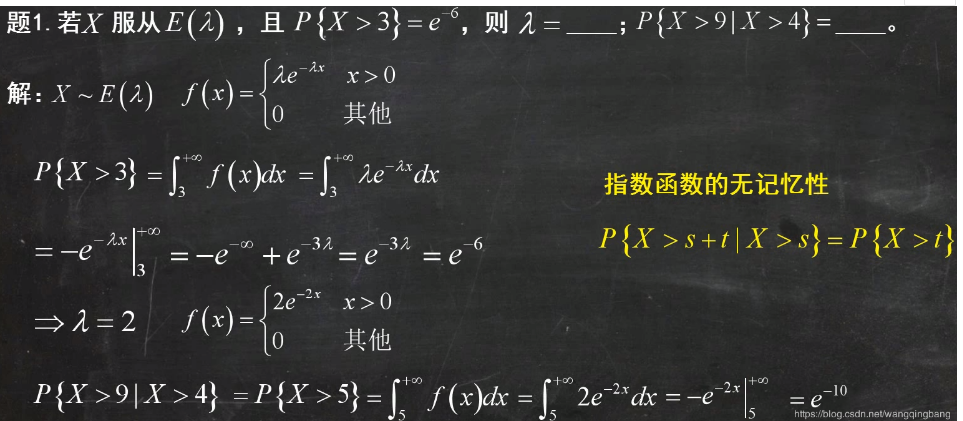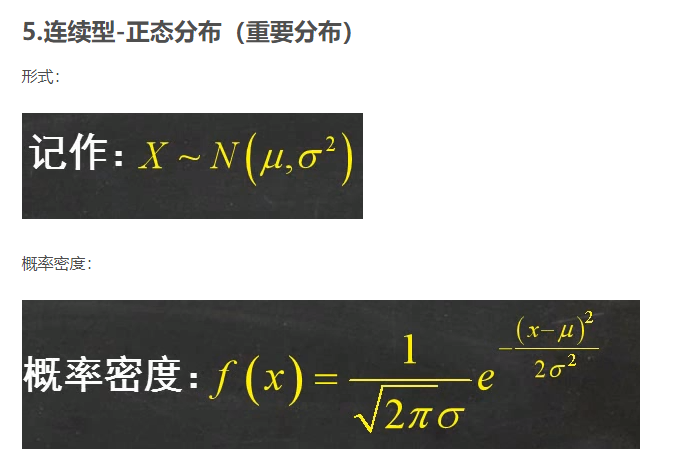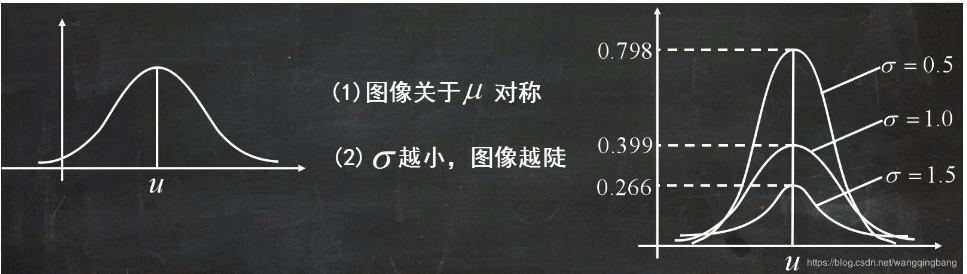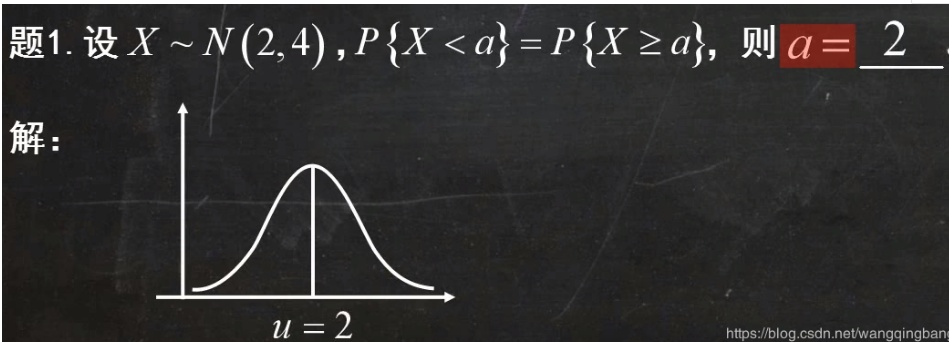对称性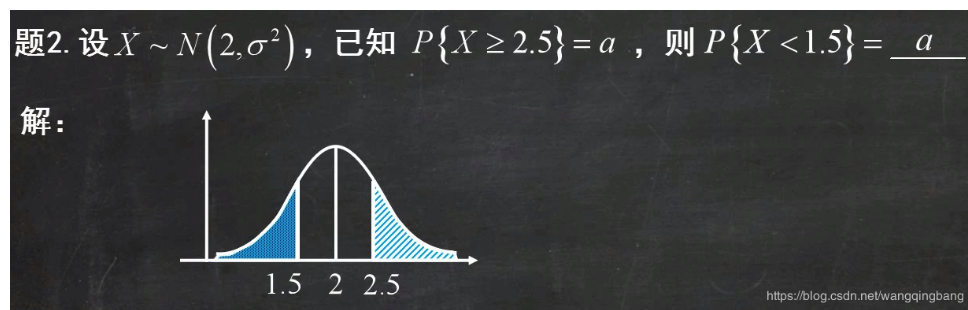理解：注意，σ 越小，则曲线越陡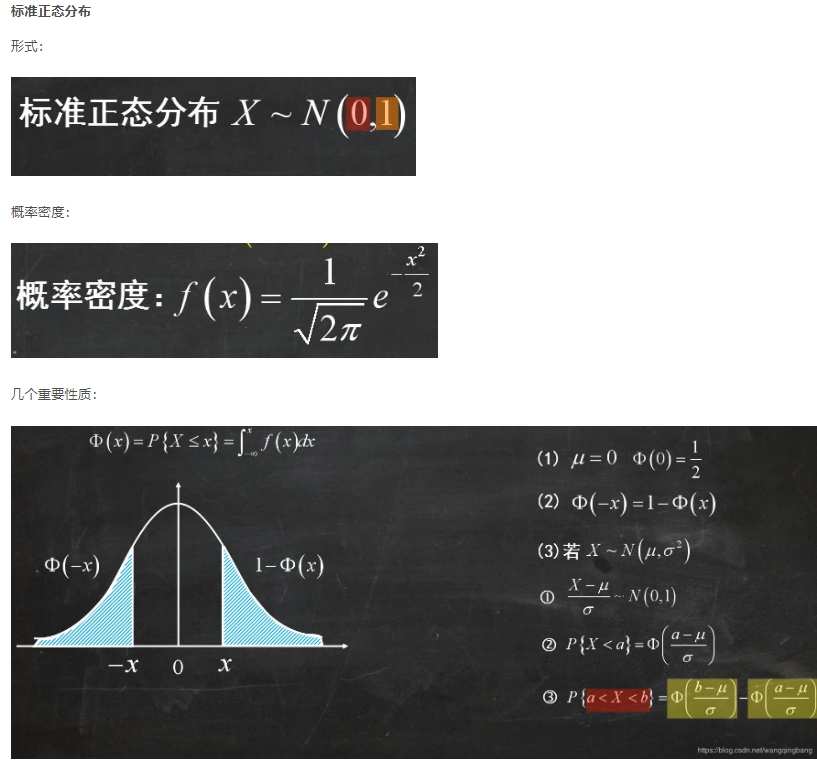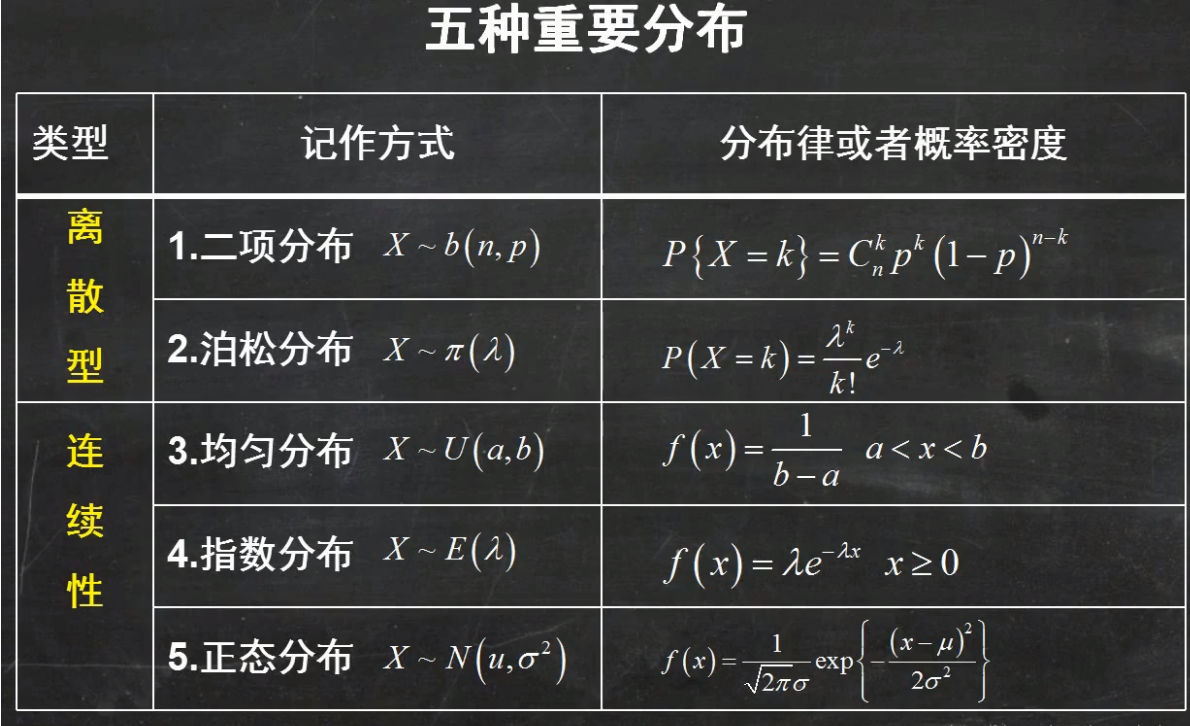展开全文• 第三章 多维随机变量及其分布 3.4相互独立的随机变量 定义： 简单说就是事件X跟Y没啥关系。故他俩一起发生的概率等于他们单独都发生的概率。 对于离散型随机变量： 例： 解： 对于连续型随机变量： 例 如图：...
• 目录第一节 离散型随机变量及其分布随机变量定义概率分布律常用离散型随机变量及其分布律分布函数定义性质 第一节 离散型随机变量及其分布 随机变量 定义 设EEE是随机试验，它的样本空间是U={e}U=\{e\}U={e}。如果...数学
• 1.随机变量及其分布函数的定义 2.离散型随机变量 定义随机变量可能取得的值是有限个或者可列无限个 概率分布列 分布函数 常见的离散性随机变量：0-1分布、二项分布和泊松分布 3.连续型随机变量 定义 分布...
• 定义随机变量为数列以重构概率论公理体系，陈必红，，目前的概率论公理对于随机变量定义是不易掌握的，例如，“任给两个随机变量”是什么意思，就不容易把握。本文重新定义了概率论
• ## 统计-随机变量

千次阅读 2018-03-03 14:29:30
设随机试验E的样本空间为S={e}，若X=X(e)是定义在样本空间S的上的一个单值实函数，则称X=X(e)为随机变量 简单说，让每一个样本点e对应着唯一的实数X(e)，便得到随机变量X=X(e) 离散型随机变量随机变量只可能...
• 定义随机变量（random variable）表示随机现象（在一定条件下，并不总是出现相同结果的现象称为随机现象）中各种结果的实质函数（一切可能的样本点）。例如某一时间内公共汽车站等车的人数，电话交换台在一定时间...
• ## 随机变量及其分布

千次阅读 2019-01-08 00:33:16
X = X(e) 是定义在样本空间S上的实值单值函数，称X=X(e)为随机变量。其中e为样本点。 例子 离散型随机变量及其规律 离散型随机变量随机变量的全部可能取到的值是有限多个或者可列无限多个。 （0-1）分布 ...
• 本文你主要讲解了随机变量，分布函数的定义及基本性质；离散型随机变量的二项分布和泊松分布；连续型随机变量定义，概率密度的性质，以及主要的均匀分布，正态分布，标准正态分布，Γ分布和指数分布。
• ## 随机变量

千次阅读 2019-09-18 17:05:26
一、中心问题：将实验结果数量化 二、随机变量 ...2.1、常见的两类随机变量（离散型...2.1.1、离散型随机变量定义： 若随机变量X的取值为有限个或可数 离散, 则称X为离散型随机变量。 2.2、0-1分布 ...
• 主要内容更多系列视频概率微课：第二章(1) 随机变量定义概率微课：第二章(2) 离散型随机变量概率微课：第二章(3) 两点分布及伯努利试验概率微课：第二章(4) 二项分布1概率微课：第二章(5) 二项分布2_例题讲解概率...
• 随机变量序列，也就是随机变量形成的序列。有时候为了简称，省略了变量二字。 随机变量：表示随机现象（在一定条件下,并不总是出现相同结果的现象称为随机现象）各种结果的变量（一切可能的样本点）。随机序列：随机...
• 二维随机变量的分布的解法最核心的莫过于分布函数定义法，但根据二维随机变量的类型，又有列表法、线段法、公式法（卷积公式）、面积法、全概率公式法！列表法、线段法：适用于两个离散型的二维随机变量分布的求解...
• 本章将介绍两类随机变量及描述随机变量统计规律性的分布. 第一节 随机变量
• 设随机试验的样本空间为 S={e}S = \{e\}S={e} ，X=X(e)X = X_{(e)}X=X(e)​是定义在样本空间SSS上的实值单值函数，称X=X(e)X = X{(e)}X=X(e)为随机变量 随机变量与普通变量有何不同 随机变量的取值随试验结果而定，...
• 首先，由高斯随机变量定义可得其概率密度为： 此时，令 即进行如下所示的的坐标变换（即极坐标变换）： 由该坐标变换可将原概率密度变换为： 若设R是Rayleigh随机变量，Θ是（0,2pi）均匀分布随机变量。 那么...c++
• 3.1 二维随机变量 文章目录3.1 二维随机变量二维随机变量及其分布函数二维离散型随机变量二维连续型随机变量 ...这个定义是通用的，无论是离散型随机变量还是连续型随机变量都适用。 用图形解释就是...
• 1.随机变量定义 2.离散随机变量分类 3.连续随机变量分类 1 随机变量 随机变量是样本空间点的函数，X=F(e)X=F(e)X=F(e). 离散随机变量：可数或无限可列 2离散随机变量分类 2.1 0-1分布 随机变量X的取值为1和0...
• 本文为不确定的随机变量提出了一个交叉熵的新定义，并推导了一个公式。 此外，本文介绍了不确定随机变量的广义交叉熵，并讨论了其性质。 基于交叉熵的定义，提出了度约束最小生成树（DCMST）问题的交叉熵机会分布...
• 二维随机变量与二维离散型随机变量问题引入定义例二维随机变量的分布函数定义二维随机变量分布函数的几何意义二维随机变量分布函数的计算二维随机变量分布函数的性质例二维离散型随机变量定义例例 问题引入 ...
• 随机变量的分布 连续性随机变量 离散型随机变量 随机变量 实验中的各种统计的数值 离散型随机变量 ...随机变量并不是连续变化...为离散型的随机变量定义的 本身为概率值, X是随机变量的取值,P为概率值 离散型...
• 2.1 随机变量 开宗明义，定义先行。作为第二章的第一小节，他的主要任务就是去讲为什么要有随机变量和什么是随机变量。 先来看为什么要有随机变量： 引入随机变量按我的理解就是为了把语文转化为数学，即把用语言去...
• 定义：设E是一个随机试验，它的样本空间是S={e}，设X=X(e)和Y=Y(e)，称(X,Y)为二维随机变量 1、二维离散型随机变量及分布律 (1)、设二维离散型随机变量(X,Y)的所有可能取的值为(Xi,Yj)，则其分布律为： 也可以表示......